Himanshi Singh — Published On March 26, 2021

## Introduction

In the previous article, we saw the Chi-Square algorithm- How to select Best Split in Decision Trees using Chi-Square. In this article, we will look at one more algorithm to help us decide the right split in decision trees. This algorithm is Information gain.

Note: If you are more interested in learning concepts in an Audio-Visual format, We have this entire article explained in the video below. If not, you may continue reading.

Let’s begin!

Consider these three nodes. Can you guess which of these nodes will require more information to describe them?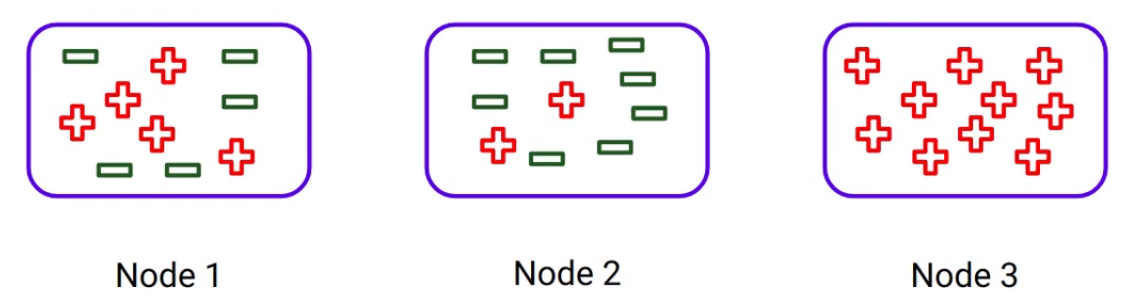As you can see here, Node 3 is a pure node since it only contains one class. Whereas Node 2 is less pure and Node 1 is the most impure node out of all these three since it has a mixture of classes. And the percentage distribution in Node 1 is 50%. So as the impurity of the node increases, we require more information to describe them. And that’s why Node 1 will require more information as compared to the other nodes.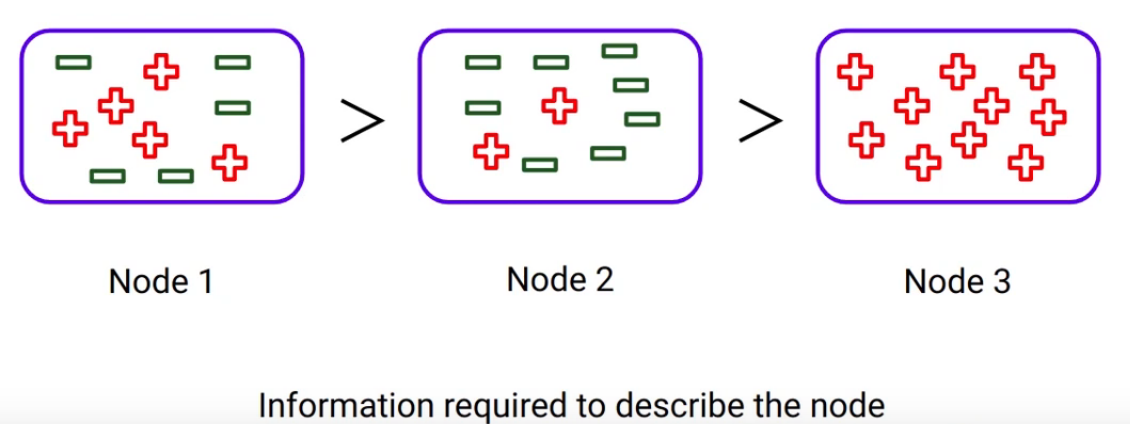So we can say that more impure nodes require more information to describe. Let’s continue an example. Look at the split, look at the right-hand side especially-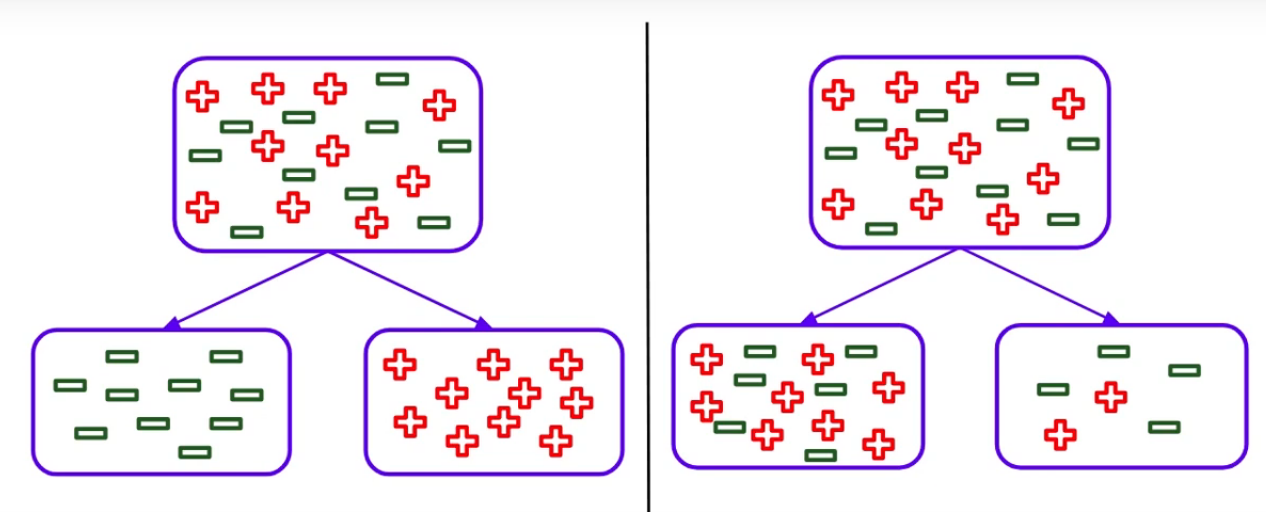It is leading to less homogeneous nodes compared to the split on the left, which is producing completely homogeneous nodes. What can you infer from that? Split on the right is giving less information gain. So we can say that a higher information gain leads to more homogeneous or pure nodes. The information gain can be calculated as: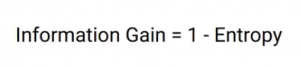But wait, what is Entropy here? How can we calculate that? Let’s look at how we can do that.

We can calculate the entropy as: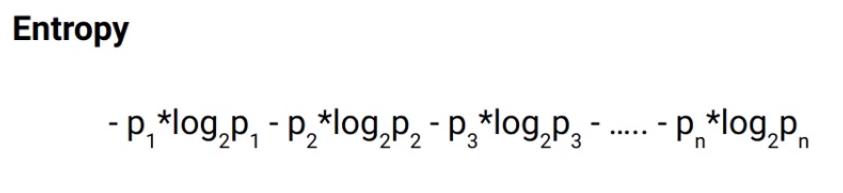Here P1, P2, and P3 are the percentages of each class in the node. Consider this node as an example-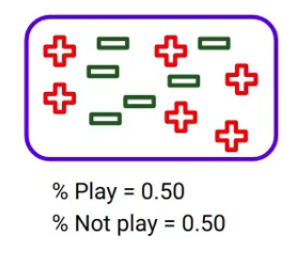Here the percentage of students who play cricket is 0.5 and the percentage of students who do not play cricket is of course also 0.5

Now if I use the formula to calculate the entropy: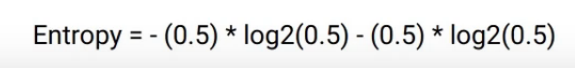The percentage of Play class is 0.5 multiplied by the log of 0.5 bases two minus the percentage of do not play, which is 0.5 multiply log of 0.5. Since the log of 0.5 bases two is -1, the entropy for this node will be 1. Let’s consider one more node-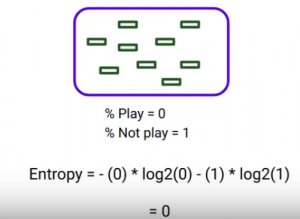You can see it’s a pure node as it only contains a single class. The percentage of students who play cricket, in this case, is 0 and who does not play is 1. So after applying the formula to these numbers, you can imagine that the entropy will come out to be zero. When you compare the entropy values here, we can see that:

Lower entropy means more pure node and higher entropy means less pure nodes.

## Properties of Entropy

Let’s now look at the properties of Entropy.

• Similar to Gini impurity and Chi-square, it also works only with the categorical target values.
• Lesser the entropy higher the information gain, which will lead to more homogeneous or pure nodes.

These two are essentially, and basically the properties of entropy.

## Steps to calculate Entropy for a Split

• We will first calculate the entropy of the parent node.
• And then calculate the entropy of each child.
• Finally, we will calculate the weighted average entropy of this split using the same steps that we saw while calculating the Gini.

The weight of the node will be the number of samples in that node divided by the total samples. In the parent note, if the weighted entropy of the child channel is greater than the parent node, we will not consider that split. As it’s returning more impure nodes compared to the parent node. Let’s use these two splits and calculate the entropy for both of the splits-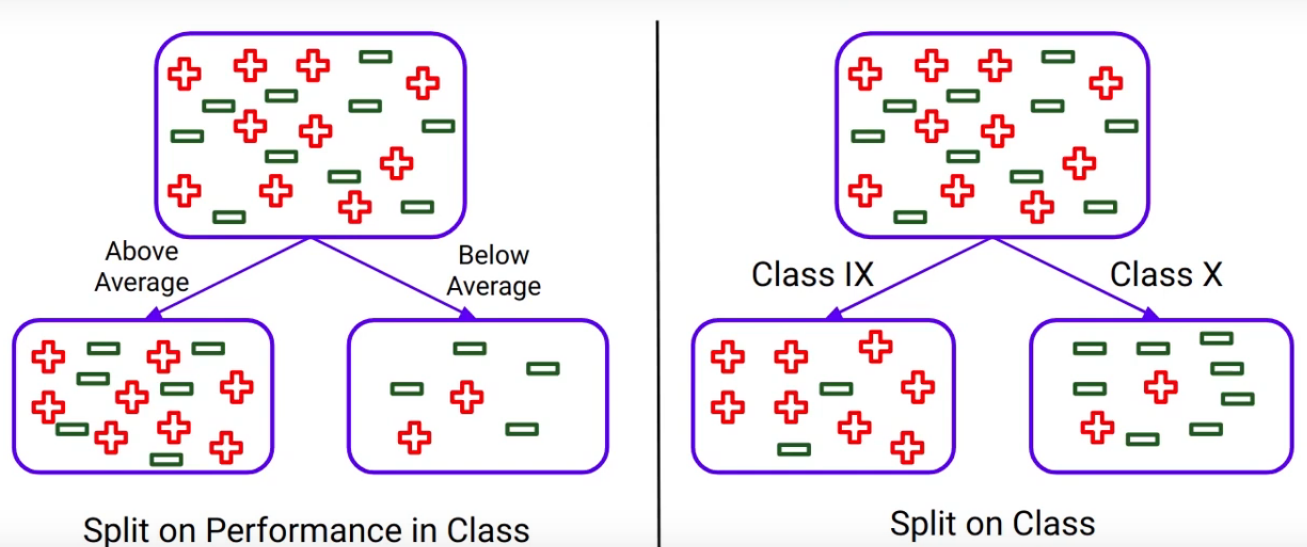Let’s start with the student performance in class. This is the split we got based on the performance that you’re very familiar with this by now-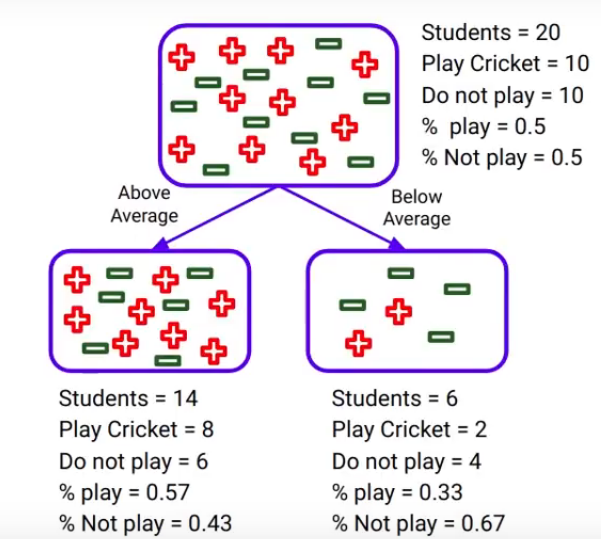the first step is to calculate the entropy for the parent. Now, the probability of playing and not playing cricket is 0.5 each, as 50% of students are playing cricket. So the entropy for the parent node will be as shown here: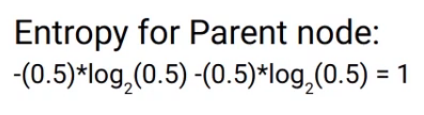and it comes out to be 1. Similarly for the sub-node “Above average”, the probability of playing is 0.57, and the probability of not playing is 0.43. When you plug in those values: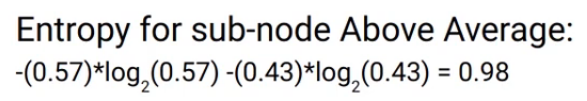The entropy comes out to be 0.98. For the sub-node “Below average”, we do the same thing, the probability of playing is 0.33 and of not playing is 0.67. And when we plug those values into the formula, we get: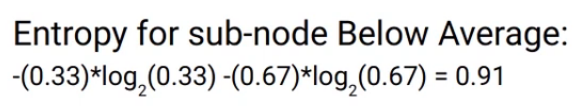Finally, the weighted entropy for the split on performance in class will be the sum of the weight of that node multiplied by the entropy of that node-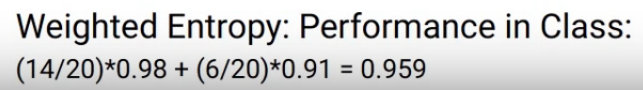The performance in class entropy will be around 0.959. Here we can see that this weighted entropy is lower than the entropy of the parent node. The parent node is 1 and the weighted entropy is 0.95. So we can say that it is returning more pure nodes compared to the parent node.

Similarly, we can calculate the entropy for “the split on Class” using the same steps that we just saw: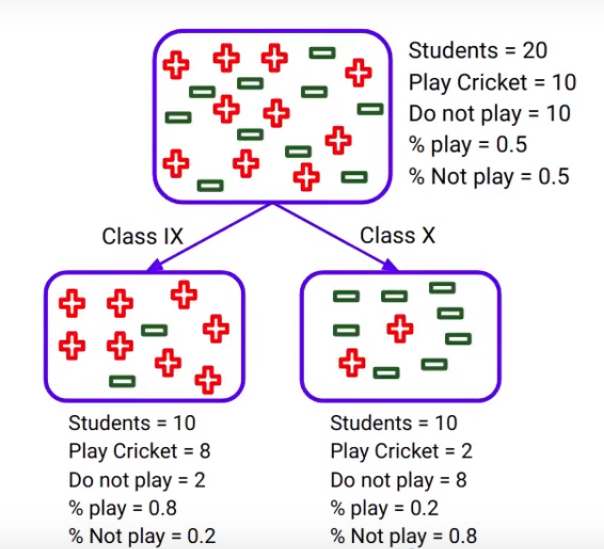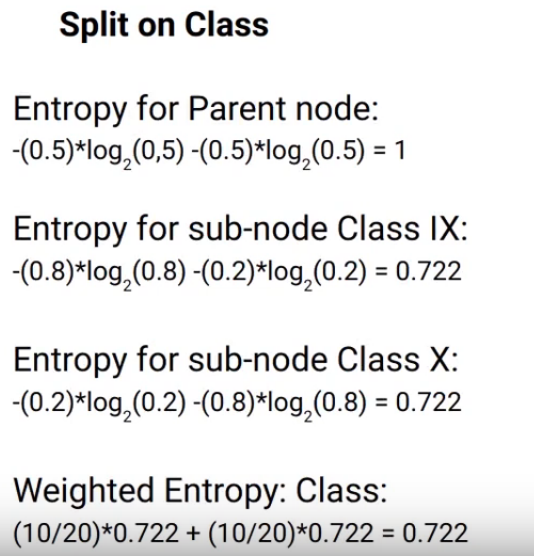Here we have calculated all the values. I’d advise you to calculate them on your own. Take a pen and paper, plug-in these values into the formula, and compare them with these results.

The weighted entropy for the split on “the Class” variable comes out with 0.722. Now we will compare the entropies of two splits, which are 0.959 for “Performance in class” and 0.722 for the split on “the Class” variable. Information Gain is calculated as:Remember the formula we saw earlier, and these are the values we get when we use that formula-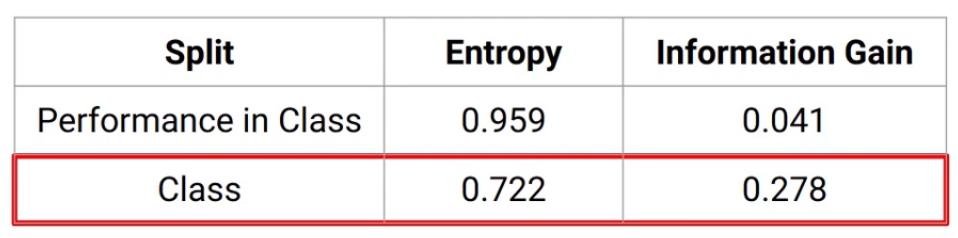For “the Performance in class” variable information gain is 0.041 and for “the Class” variable it’s 0.278. Lesser entropy or higher Information Gain leads to more homogeneity or the purity of the node. And hence split on the Class variable will produce more pure nodes.

And this is how we can make use of entropy and information gain to decide the best split.

## End Notes

In this article, we saw one more algorithm used for deciding the best split in the decision trees which is Information Gain. This algorithm is also used for the categorical target variables.

If you are looking to kick start your Data Science Journey and want every topic under one roof, your search stops here. Check out Analytics Vidhya’s Certified AI & ML BlackBelt Plus Program

If you have any queries let me know in the comment section!